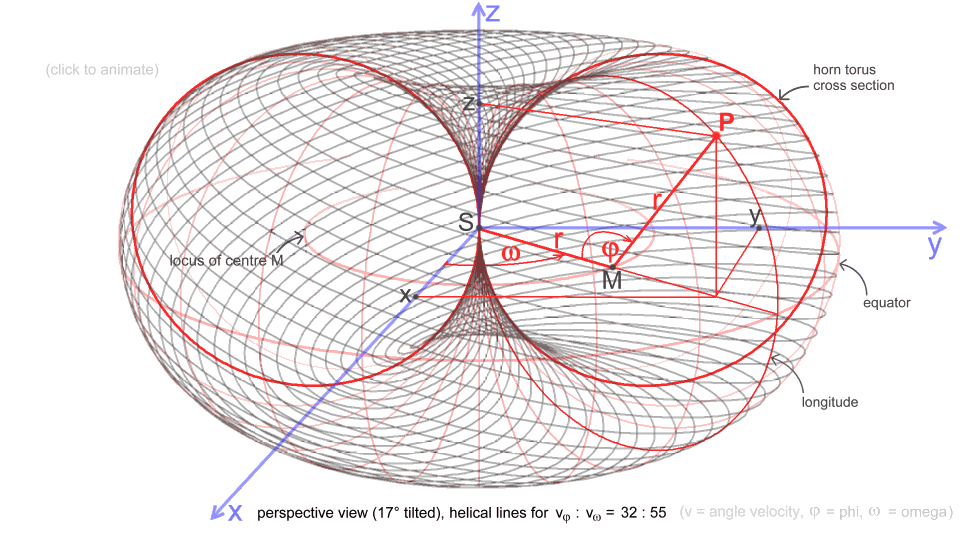index     sitemap     textscoordinates for  this animation  and its parametric form (source) : for all points P on the surface of a horn torus with fixed radius r is validx = r·(1 − cosφ)·cosω y = r·(1 − cosφ)·sinω  z = r·sinφ   the 'unrolling line', indicated in the animation, is divided into two parts (referring to the standard dynamic horn torus as unit):  r > 1 and r < 1  case r > 1: r and ω increase with φ, starting with φ1 = 2π, according r = φ / 2π (↝ r1 = 1) and ω = r·φ = φ2 / 2π (↝ ω1 = 2π), so we have x = (1 − cosφ)·cos(φ2/2π)·φ/2π y = (1 − cosφ) · sin(φ2/2π)·φ/2π z = sinφ · φ/2π(helical lines - at φ = const. each - not to scale),  see also  case r < 1: